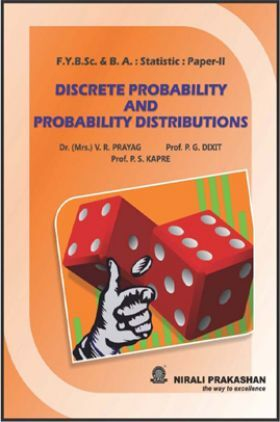# Discrete Probability And Probability Distributions Statistics Paper - II

Sold ( 54 times )
2369 Views
MRP : ₹240.00
Price : ₹159.60
You will save : ₹80.40 after 34% Discount
Inclusive of all taxes
INSTANT delivery: Read it now on your device

Save extra with 2 Offers

Get ₹ 50

Instant Cashback on the purchase of ₹ 400 or above

Product Specifications

 Publisher Nirali Prakashan All B.Sc. - Bachelor of Science books by Nirali Prakashan ISBN 9789383073788 Author: Dr. (Mrs.) V. R. Prayag, Prof. P. G. Dixit, Prof. P. S. Kapre Number of Pages 321 Available Available in all digital devicesDiscrete Probability And Probability Distributions Statistics Paper - II by Dr. (Mrs.) V. R. Prayag, Prof. P. G. Dixit, Prof. P. S. Kapre
Book Summary:

The main purpose of the book is to provide foundation as well as comprehensive background of 'Discrete Probability Theory' to beginners in simple and interesting manner. In order to make the contents of the book easier to comprehend, we have included a requisite number of illustrations, remarks, figures, diagrams etc. to elucidate statistical concepts. Application of Statistics in real life situations is emphasized through illustrative examples. Ample number of graded problems, theoretical as well as numerical, are provided at the end of each chapter along with hints and answers. The numerical problems will also be useful for the F.Y.B.Sc. students to prepare for Paper – III : Practicals. A list of practicals is given in the syllabus. Appendix A compiles some important mathematical results which are needed during the entire course. Values of individual terms of binomial probabilities are given in Appendix B. A specimen paper is set for student's self assessment.

Audience of the Book :
This book Useful for B.Sc. students.

1 Sample Space and Events

2 Probability

3 Conditional Probability and Independence

4 Univariate Probability Distribution

5 Mathematical Expectation (Univariate)

6 Standard Discrete Probability Distributions : Finite Sample Space

7 Standard Discrete Probability Distributions : Countably Infinite Sample Space

8 Bivariate Discrete Probability Distribution

9 Mathematical Expectation (Bivariate)